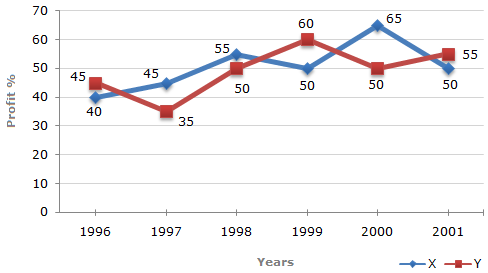# Data Interpretation - Line Charts - Discussion

Discussion Forum : Line Charts - Line Chart 4 (Q.No. 3)
Directions to Solve

The following line graph gives the percent profit earned by two Companies X and Y during the period 1996 - 2001.

Percentage profit earned by Two Companies X and Y over the Given Years

 %Profit = Income - Expenditure x 100 Expenditure3.
If the expenditures of Company X and Y in 1996 were equal and the total income of the two Companies in 1996 was Rs. 342 crores, what was the total profit of the two Companies together in 1996 ? (Profit = Income - Expenditure)
Rs. 240 crores
Rs. 171 crores
Rs. 120 crores
Rs. 102 crores
Explanation:

Let the expenditures of each companies X and Y in 1996 be Rs. x crores.

And let the income of Company X in 1996 be Rs. z crores.

So that the income of Company Y in 1996 = Rs. (342 - z) crores.

Then, for Company X we have:

 40 = z - x x 10040 = z - 1x = 100z .... (i) x 100 x 140

Also, for Company Y we have:

 45 = (342 - z) x 10045 = (342 - z) - 1x = (342 - z) x 100 .... (ii) x 100 x 145

From (i) and (ii), we get:

 100z = (342 - z) x 100z = 168. 140 145

Substituting z = 168 in (i), we get : x = 120.Total expenditure of Companies X and Y in 1996 = 2x = Rs. 240 crores.

Total income of Companies X and Y in 1996 = Rs. 342 crores.Total profit = Rs. (342 - 240) crores = Rs. 102 crores.

Discussion:
19 comments Page 2 of 2.

Santosh said:   6 years ago
Nice @Abhi.

Amit said:   7 years ago
Thank you. Nice explanation @Abhi.

Shailesh mishra said:   7 years ago
Thanks for the explanation.

Mani deepthi reddy said:   7 years ago
Thanks for the explanation of the answer.

Abhi said:   8 years ago
145x/100+140x/100 = 342.

x = 120.

45+40 = 85%of 120 = 102.

I did not understood this question can anyone simply it in easy way.

Sivaraj said:   9 years ago
Is it possible to solve while examination with in time?

Anjali Gupta said:   9 years ago
40/100 = z/x-1 = 100z/140.

100z/140.
How is come?